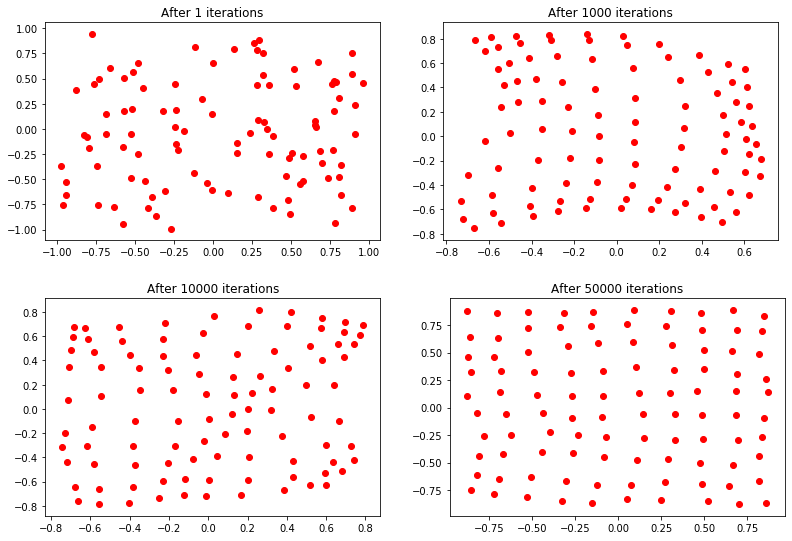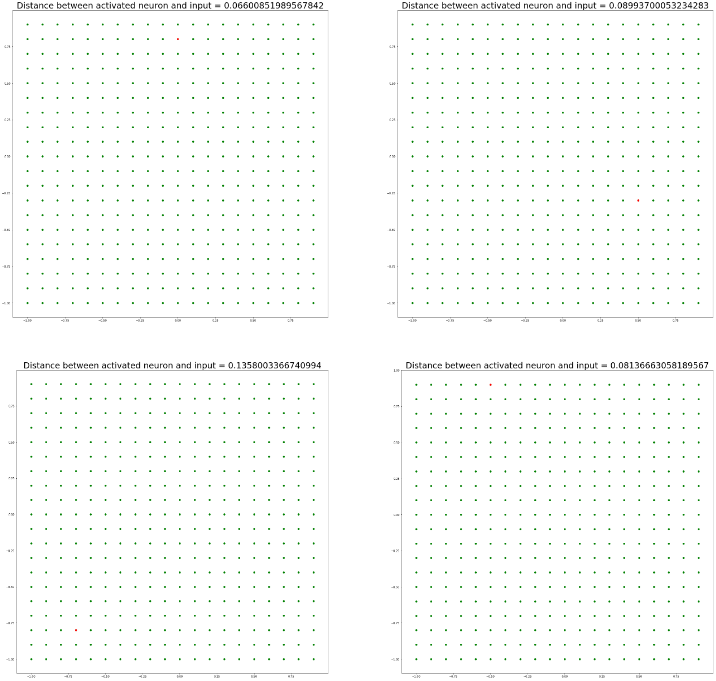Related Articles
ANN – Implementation of Self Organizing Neural Network (SONN) from Scratch
• Last Updated : 10 Jul, 2020

To implement a SONN, here are some essential consideration-

1. Construct a Self Organizing Neural Network (SONN) or Kohonen Network with 100 neurons arranged in a 2-dimensional matrix with 10 rows and 10 columns
2. Train the network with 1500 2-dimensional input vectors randomly generated in the interval between -1 and +1
3. Select initial synaptic weights randomly in the same interval -1 and +1
4. Assign learning rate parameteris equal to 0.1
5. Objective is to classify 2-dimensional input vectors such that each neuron in the network should respond only to the input vectors occurring in its region
6. Test the performance of the self organizing neurons using the following Input vectors:Python Implementation of SONN:

 # Importing Libraries import mathfrom tqdm.notebook import tqdmimport matplotlib.pyplot as pltimport numpy as np  # Generating Data : using uniform random number generator data_ = np.random.uniform(-1, 1, (1500, 2))# print(data_.shape)  # Hyperparameter Initializationx, y = 10, 10        # dimensions of Mapsigma = 1.           # spread of neighborhoodlearning_rate = 0.5  # learning rateepochs = 50000       # no of iterationsdecay_parameter = epochs / 2   # decay parameter  # Activation map and Assigning Weights# using random number generation activation_map = np.zeros((x, y))weights = 2 * (np.random.ranf((x, y, data_.shape)) - 0.5)# print(weights.shape)  # Define Neighborhood Regionneighbour_x = np.arange(x)neighbour_y = np.arange(y)  # Function: decay_learning_rate_sigmadef decay_learning_rate_sigma(iteration):  learning_rate_ = learning_rate/(1 + iteration / decay_parameter)  sigma_ = sigma / (1 + iteration / decay_parameter)    return learning_rate_, sigma_  # Function: to get winner neurondef get_winner_neuron(x):  s = np.subtract(x, weights) # x - w  it = np.nditer(activation_map, flags =['multi_index'])  while not it.finished:      # || x - w ||      activation_map[it.multi_index] = np.linalg.norm(s[it.multi_index])        it.iternext()    return np.unravel_index(activation_map.argmin(), activation_map.shape)  # Update weightsdef update_weights(win_neuron, inputx, iteration):  # decay learning rate and sigma  learning_rate_, sigma_ = decay_learning_rate_sigma(iteration)    # get neighborhood about winning neuron (Mexican hat function)  d = 2 * np.pi * (sigma_**2)  ax = np.exp(-1 * np.square(neighbour_x - win_neuron) / d)  ay = np.exp(-1 * np.square(neighbour_y - win_neuron) / d)    neighborhood = np.outer(ax, ay)    it = np.nditer(neighborhood, flags = ['multi_index'])  while not it.finished:      weights[it.multi_index] += learning_rate_ * neighborhood[it.multi_index]                                 * (inputx - weights[it.multi_index])      it.iternext()  # Training model: Learning Phasefor epoch in tqdm(range(1, epochs + 1)):  np.random.shuffle(data_)  idx = np.random.randint(0, data_.shape)  win_neuron = get_winner_neuron(data_[idx])  update_weights(win_neuron, data_[idx], epoch)    if epoch == 1 or epoch == 100 == 0 or epoch == 1000 or      epoch == 10000 or epoch == 50000:    plot_x = []    plot_y = []      for i in range(weights.shape):      for j in range(weights.shape):        plot_x.append(weights[i][j])        plot_y.append(weights[i][j])      plt.title('After ' + str(epoch) + ' iterations')    plt.scatter(plot_x, plot_y, c = 'r')    plt.show()    plt.close()

Output:# Testing the model performancetest_inputs = np.array([[0.1, 0.8], [0.5, -0.2], [-0.8, -0.9], [-0.6, 0.9]]) # print(test_inputs.shape)   # The plots below depict the working of this Kohonen Network on # given test inputs [0.1, 0.8], [0.5, -0.2], [-0.8, -0.9], [-0.6, 0.9]for i in range(test_inputs.shape):  test_input = test_inputs[i, :]  win_neuron = get_winner_neuron(test_input)    plot_x = np.arange(-1, 1, 0.1)  plot_y = np.arange(-1, 1, 0.1)  xx, yy = np.meshgrid(plot_x, plot_y)    coordx, coordy = weights[win_neuron][win_neuron],                            weights[win_neuron][win_neuron]  dist = math.sqrt((coordx-test_input)**2 + (coordy - test_input)**2)  coordx = round(coordx, 1)  coordy = round(coordy, 1)    plt.figure(figsize =(20, 20))  plt.title("Distance between activated neuron and input = " +             str(dist), fontsize = 30)  plt.scatter(xx, yy, c = 'g')  plt.scatter(coordx, coordy, c = 'r')  plt.show()  plt.close()
Output:All the 10×10 neurons are represented by green color. The nearest neuron which is activated by the test input is shown with red color. Here, the euclidean distances between activated neurons and test inputs are also shown in these plots. Respective neuron will respond for each of the test input vector samples.My Personal Notes arrow_drop_up